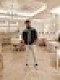Question: What is the drawback of Double DES algorithm? How is it overcome by Triple DES?
0
mumbai university • 592 views
 modified 3 months ago  • written 3 months ago byneelchheda45 • 0
0
• Consider a message 'n' Which is to the encrypted
• The corresponding block cipher for one application of the DES applications is represented by Ek where K is the corresponding DES key
• The output of 2-DES is C = Ek2 ( E (m)) to decrypt similarly, m = Dk1(Dk2(C))
• The cipher 2-DES should offer additional security, equivalent to both K1 & K2.
• The cipher 2-DES obtained by the repeated application of DES is called 2DES = DES X DES. This is called a product cipher obtained by the composition of two cipher such an idea can similarly by extended to multiple ciphers
• The composition of two ciphers with two different keys cannot be obtained by a single application of DES with a key
• This 2DES is encrypted to provide security equivalent to 56 X 12 = 112 bits
• But such a cipher can be attached by an attach method which is called meet in the middle ( MIM ) attach
• As double DES , DES has a problem of the MIM attach, triple DES was developed
• The expected security of 3-DES is 112 bitsTriple DES

• There are in general two flavors of 3-DES & two ways for implementation of 3-DES
• The first implementation uses three keys K1,K2,K3 the ciphertent of 'm' is thus obtained by

C = DESK1[DESk2 ( DESK3 (m))]The second way to implement 3-DES is using two keys, thus

C = DESK1[DES-1k2 ( DESK3 (m))]

 written 3 months ago byneelchheda45 • 0